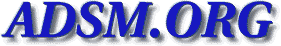Veritas-bu [Top] [All Lists]

## [Veritas-bu] LTO GEN 2 L180

2003-07-18 20:17:21
 Subject: [Veritas-bu] LTO GEN 2 L180 Karl.Rossing AT Federated DOT CA (Karl.Rossing AT Federated DOT CA) Fri, 18 Jul 2003 19:17:21 -0500
 ```This is a multipart message in MIME format. --=_alternative 0001C5A486256D68_= Content-Type: text/plain; charset="ISO-8859-1" Content-Transfer-Encoding: quoted-printable Kim, I just went threw this this morning. 1) You need the SCSI card drivers from SUN Solaris 8 http://sunsolve.sun.com/pub-cgi/retrieve.pl?patchid=3D108725&collection=3Df= patches&mode=3Dresults Solaris 9 http://sunsolve.sun.com/pub-cgi/retrieve.pl?patchid=3D113277&collection=3Df= patches&mode=3Dresults 2) I was running solaris 8 so i also needed the latest sg driver patch. I=20 was able to get that using the "latest recomended and security patch" http://sunsolve.sun.com/pub-cgi/show.pl?target=3Dpatchpage 3) I updated my OBP to the latest rev 4) It's a dual channel card, for some reason, i was getting a parity error = when i had my L8(lto2) connected to channel 2 and nothing to channel 1. Now i gotta tune my backup cuz to me it seems slow. Maybe we can compare=20 performance numbers later. I hope i helped. Karl-Fr=E9d=E9ric R=F6ssing Technical Analyst Federated Insurance Companies of Canada http://www.federated.ca maintenant disponible en Fran=E7ais veritas-bu-admin AT mailman.eng.auburn DOT edu wrote on 07/18/2003 06:53:45 PM: >=20 > I have currently scsi3(X6758, sun part#375-3057) hba > connects L180 tape drives (4 HP LTO GEN2). robot is > connect to E420 on board se. at the ok promt, I can > run probe-scsi-all and see all the tape drives. if I > run sgscan, I can't not see any drive at run level 3. > I also put tapes in the drive and use mt command, it > shows "HP Ultrim 2-scsi" I am assuming sol8 reconize > lto gen 2. why veritas netbackup can't see the tape > drive(s)?=20 > netbackup 4.5 FP3. >=20 > =5F=5F=5F=5F=5F=5F=5F=5F=5F=5F=5F=5F=5F=5F=5F=5F=5F=5F=5F=5F=5F=5F=5F=5F= =5F=5F=5F=5F=5F=5F=5F=5F=5F=5F > Do you Yahoo!? > SBC Yahoo! DSL - Now only \$29.95 per month! > http://sbc.yahoo.com > =5F=5F=5F=5F=5F=5F=5F=5F=5F=5F=5F=5F=5F=5F=5F=5F=5F=5F=5F=5F=5F=5F=5F=5F= =5F=5F=5F=5F=5F=5F=5F=5F=5F=5F=5F=5F=5F=5F=5F=5F=5F=5F=5F=5F=5F=5F=5F > Veritas-bu maillist - Veritas-bu AT mailman.eng.auburn DOT edu > http://mailman.eng.auburn.edu/mailman/listinfo/veritas-bu >=20 --=_alternative 0001C5A486256D68_= Content-Type: text/html; charset="ISO-8859-1" Content-Transfer-Encoding: quoted-printable
Kim,

I just went threw this this morning.=

1) You need the SCSI card drivers fr= om SUN
Solaris 8
http://sunsolve.sun.com/pub-cgi/retr= ieve.pl?patchid=3D108725&collection=3Dfpatches&mode=3Dresults
Solaris 9
http://sunsolve.sun.com/pub-cgi/retr= ieve.pl?patchid=3D113277&collection=3Dfpatches&mode=3Dresults

2) I was running solaris 8 so i also needed the latest sg driver patch. I was able to get that using the "l= atest recomended and security patch"
http://sunsolve.sun.com/pub-cgi/show= .pl?target=3Dpatchpage
3) I updated my OBP to the latest re= v
4) It's a dual channel card, for some reason, i was getting a parity error when i had my L8(lto2) connected to channel 2 and nothing to channel 1.

Now i gotta tune my backup cuz to me it seems slow. Maybe we can compare performance numbers later.

I hope i helped.

Karl-Fr=E9d=E9ric R=F6ssing
Technical Analyst
http://www.federated.ca maintenant disponible en Fran=E7ais

veritas-bu-admin AT mailman.eng.auburn DOT edu wrote on 07/= 18/2003 06:53:45 PM:

>
> I have currently scsi3(X6758, sun part#375-3057) hba
> connects L180 tape drives (4 HP LTO GEN2). robot is
> connect to E420 on board se.  at the ok promt, I can
> run probe-scsi-all and see all the tape drives. if I
> run sgscan, I can't not see any drive at run level 3.
> I also put tapes in the drive and use mt command, it
> shows "HP Ultrim 2-scsi" I am assuming sol8 reconize
> lto gen 2. why veritas netbackup can't see the tape
> drive(s)?
> netbackup 4.5 FP3.
>
> =5F=5F=5F=5F=5F=5F=5F=5F=5F=5F=5F=5F=5F=5F=5F=5F=5F=5F=5F=5F=5F=5F=5F= =5F=5F=5F=5F=5F=5F=5F=5F=5F=5F=5F
> Do you Yahoo!?
> SBC Yahoo! DSL - Now only \$29.95 per month!
> http://sbc.yahoo.com
> =5F=5F=5F=5F=5F=5F=5F=5F=5F=5F=5F=5F=5F=5F=5F=5F=5F=5F=5F=5F=5F=5F=5F= =5F=5F=5F=5F=5F=5F=5F=5F=5F=5F=5F=5F=5F=5F=5F=5F=5F=5F=5F=5F=5F=5F=5F=5F
> Veritas-bu maillist  -  Veritas-bu AT mailman.eng.auburn DOT edu
> http://mailman.eng.auburn.edu/mailman/listinfo/veritas-bu
>
--=_alternative 0001C5A486256D68_=-- ```
 Current Thread [Veritas-bu] LTO GEN 2 L180, kim lee [Veritas-bu] LTO GEN 2 L180, Karl.Rossing AT Federated DOT CA <=